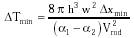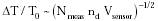Nanomedicine, Volume I: Basic Capabilities

Robert A. Freitas Jr., Nanomedicine, Volume I: Basic Capabilities, Landes Bioscience, Georgetown, TX, 1999

4.6.3 Thermal-Expansion Temperature Sensors

Consider a thin rod of length x and width w, constructed as a sandwich of two dissimilar materials each of thickness h, having coefficients of linear expansion a1 and a2, respectively (Fig. 4.8). When heated, the two materials expand differentially to lengths of x (1 + ai DT) (neglecting the quadratic and higher-order terms in ai), producing a cantilever deflection of qt = (x/h) (a1 - a2) (radians) from simple geometry. The minimum detectable temperature change DTmin = 2 p Dxmin / x qt or{Eqn. 4.37}

where volume of the sandwich rod Vrod = 2 h w x. Taking Dxmin = 10 pm, a1 = 2.38 x 10-5 /K for aluminum,460 a2 = 8 x 10-7 /K for diamond at 310 K,460 h = w = 5 nm, and assuming sensor volume Vsensor ~ 2 Vrod in a tightly packed three-dimensional coil configuration, DTmin = 1 microkelvin for an L3 ~ (227 nm)3 sensor ~ 1% of the volume of a 1-micron nanorobot. Use of a suitable negative-coefficient material for a2, such as the ceramics zirconium tungstate1025 and cordierite, can further reduce L by 5%-10%.

Measuring the volume of the sensor is essentially like taking an inventory of total stored thermal energy, which has a kT N1/2 fluctuation term from adding the N uncorrelated kT fluctuations; hence{Eqn. 4.38}

Hence, to achieve DT ~ 1 microkelvin at T0 = 310 K with this sensor may require Nmeas ~ 47 million independent measurements of Dtmin = 1 nanosec each, or tmeas ~ 47 millisec.

Micromechanical silicon cantilever heat sensors (microcalorimeters) of ~20,000 micron3 volume have already achieved DTmin = 10 microkelvins at room temperature (DTmin / T ~ 3 x 10-8), responding to ~1 pJ of heat in a measurement time tmeas ~ 1 millisec.461 Thermal-expansion nanosensors might also exploit the temperature sensitivity of viscoelastic materials (e.g., modulus of relaxation).

Last updated on 17 February 2003